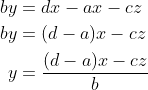## Solving a linear equation with multiple variables

A linear equation of many variables can be expressed as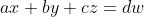Where a,b,c, and d are considered as constants and x,y,z, and w are variables.

We can solve the equation for any of the variables by following simple isolating rules

For example, to solve for y, we move to the right side of the equation those terms not containing y: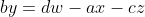Finally, we move the coefficient by dividing the above expression: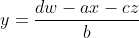There are some special cases where one of the variables of the left side is repeated in the right side:If we wanted to solve for the repeated variable, then we must simplify and then solve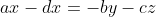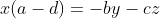If we wanted to solve for other than the repeated variable, we must simplify anyway but on the right side